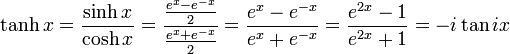# Hyperbolic function proof

## Homework Statement

Prove that:
(1+tanhx)/(1-tanhx)=e^(2x)

## Homework Equations## The Attempt at a Solution

I tried substituting tanhx for (e^x-e^(-x))/(e^x+e^(-x)) and for (e^(2x)-1)/(e^(2x)+1))

But I really have no clue how to continue...

tiny-tim
Homework Helper
Hi Pietair!Hint : (1+tanhx)/(1-tanhx) = (1 + sinhx/coshx)/(1 - sinhx/coshx) = … ?I don't know how to rewrite it to something more "common".

tiny-tim
Homework Helper

I don't know how to rewrite it to something more "common".

try simplifying (1 + sinhx/coshx)/(1 - sinhx/coshx) …

get rid of the internal fractions(coshx+sinhx)/(coshx-sinhx)

= (0.5e^x+0.5e^(-x)+0.5e^x-0.5e^(-x))/((0.5e^x+0.5e^(-x)-0.5e^x+0.5e^(-x))

= e^x/e^(-x)

= e^2x (proven)

Thanks a lot!